• Call Now

1800-102-2727•

# Convex lens, uses, practice problems, FAQs

Suman got a science project from her school for final examination. She was told to use a convex lens in her project. She can make her project on various topics of optics by using convex lenses. But first she needs to know about the various uses and application of convex lenses. In this page we will discuss various uses of convex lenses .

Table of content

• What is a convex lens ?
• Uses of convex lens
• Practice problems
• FAQs

## What is a convex lens ?

A convex lens is a curved glass plate thin at the corner and thick at the middle used to converge the rays of light.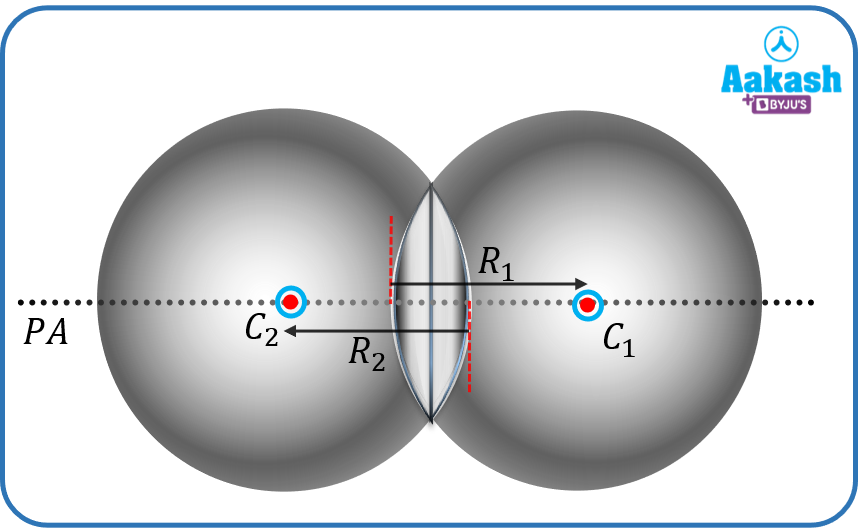The centers of the spheres from which the Convex lenses are made off are called the center of curvature (C1& C2) of the lens.The middle point of the lens is called the optical center of the lens. The distance between the center of curvature and optical center is called the radius of curvature (R1& R2). The line joining the center of curvature and optical center is called the principal axis. The point on the principle axis at which the parallel light rays converge after the refraction is called focal point and the distance of this point from the optical center is called focal length.

Lets see the uses of convex lenses.

## Uses of convex lens

The convex lens is used in various applications. Some of these are given below-

1. Magnifying glass - magnifying glass is used to see large images of an object. As we know, when an object is placed between the first principal focus and the optical center of a convex lens, then we get an enlarged, virtual and erect image. So we can use it as a magnifying glass. If we put this magnifying glass such that the light coming from the sun focuses on the paper, then it can burn the paper also.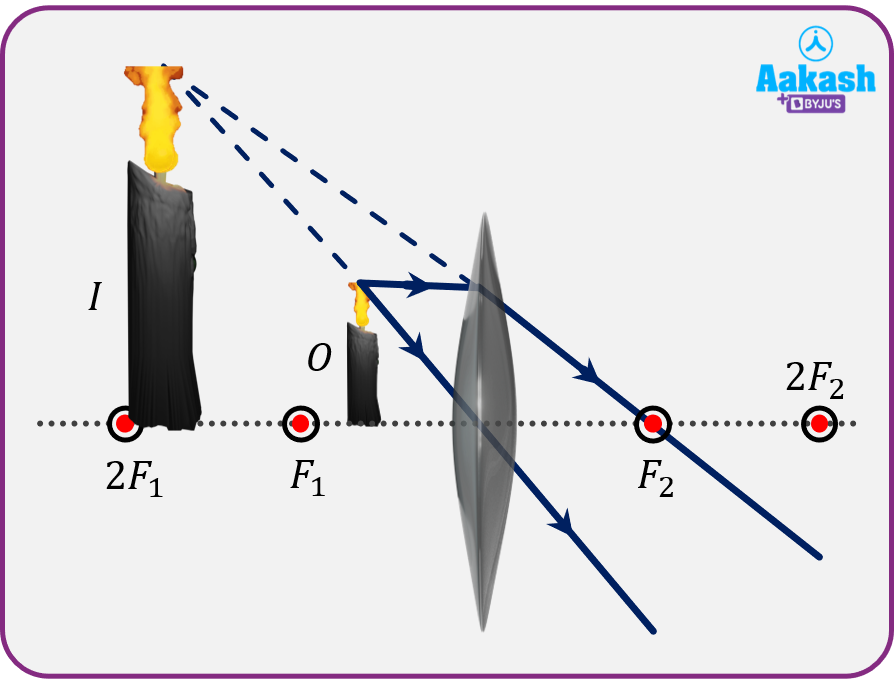1. Human Eye - In our eyes there is a natural convex lens which helps us to see the objects. This convex lens focuses the light rays to the retina. The convex lens has an ability to adjust its focal length according to the distance of the object, with the help of ciliary muscles.
1. Contact lenses- Over the period muscle in the eyes gets weaker and has difficulty adjusting the focal length, hence the light ray is focused either in front of the retina or back of the retina. These conditions are considered as defects in the eye. When the light rays focus behind the retina, then this defect is called Hypermetropia. In this defect a person cannot see the object near the eye but can see the object far away. This effect is also called farsightedness. If we use a convex lens, this will converge the light ray to focus on the retina and a better view can be achieved.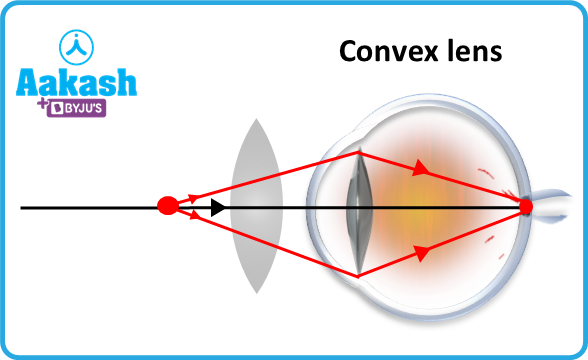1. Optical microscopes - To see the micro particles like bacteria, microfibre, microtexture surfaces etc we use optical microscopes. A microscope is nothing but just a combination of different convex lenses. As we have seen in the case of magnifying glasses, if we put an object between the focus and the optical center, then it gives the magnified image. Similarly if we use different lenses and put these lenses such that the image of first lens works as the object for the next lens, then the final image by this combination will be large in size.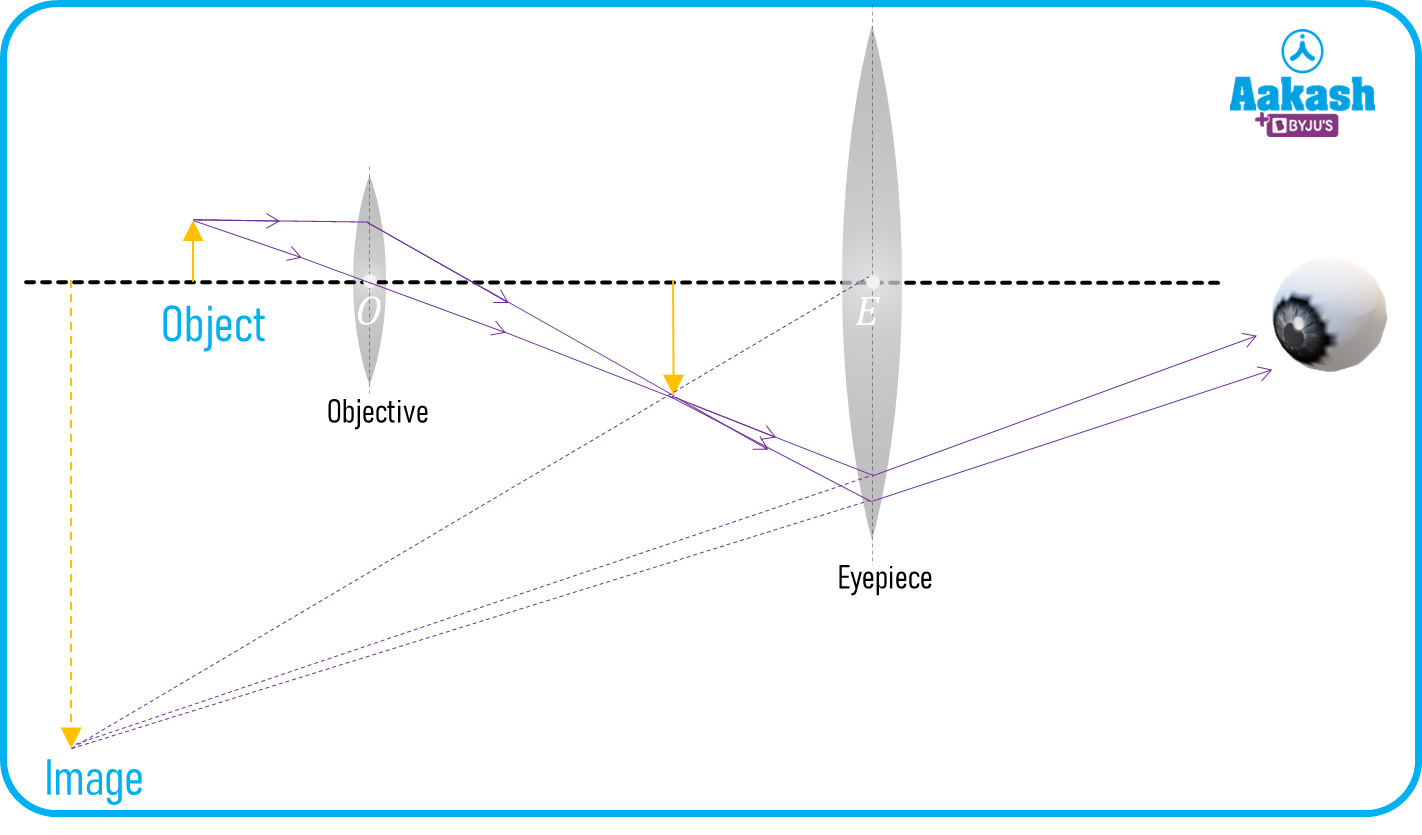1. Projector - Projectors are the optical devices which are used to project an image or video on large screens. Projectors consist of a convex lens. When a film or video is played in front of the convex lens, then a large image is projected on the screen. The image is inverted in nature. To compensate for this problem the film is inversely loaded in the projector.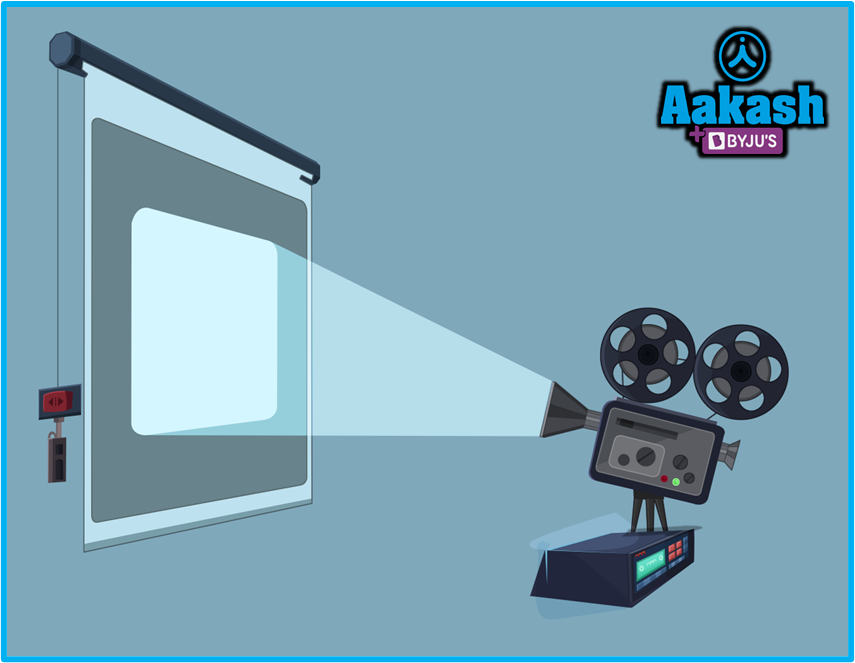1. Optical camera - Convex lenses are also used in cameras to focus an image. You have seen the photographer rotate the dial of the camera to adjust the lens. With the help of this we can get a magnified image with better quality.
1. Telescope - A telescope is an optical instrument which is used to see objects which are far away from us like satellites, planets, solar system etc. Mainly the telescope is of two types - one is reflecting telescope and other is refracting telescope. Convex lens is used in refracting telescope. The convex lens focuses the light that is coming from the object which is at a large distance. And different convex lenses magnify the image so a large image is produced.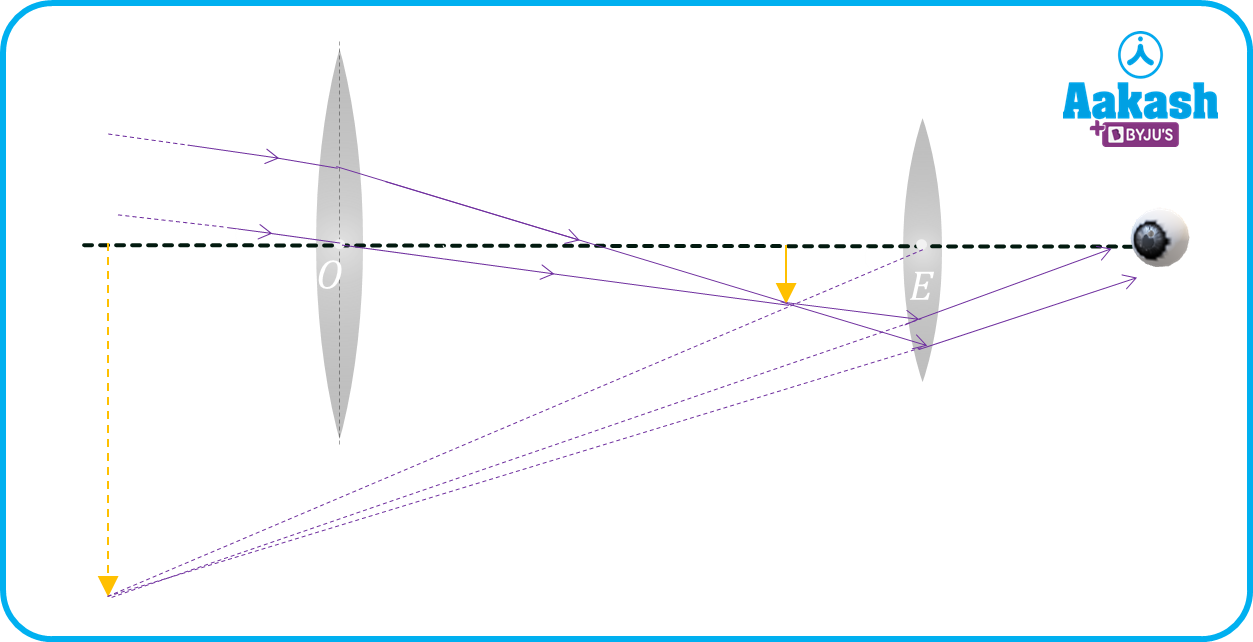1. Multi-junction solar cells - Multijunction solar cells are electric power producing devices. It has multiple p-n junctions that are made of different semiconductor materials. Convex lens is used in this as a light rays concentrator. The convex lenses are placed such that the sunlight that falls on the lens will focus on the plants which result in more power output.
1. Peep-holes - Peep holes are nothing but the small holes placed in the main doors of the house. The convex lens is inserted in this so we can view the magnified image of the person on the other side of the door. These peep holes are also used in various places for security reasons.
1. Binoculars - It is similar to the telescope but the difference is that they are used to view the object present on the earth at a large distance. Binoculars are used for the birds in the sky, to see the person on the other side of the river, used in military purposes etc.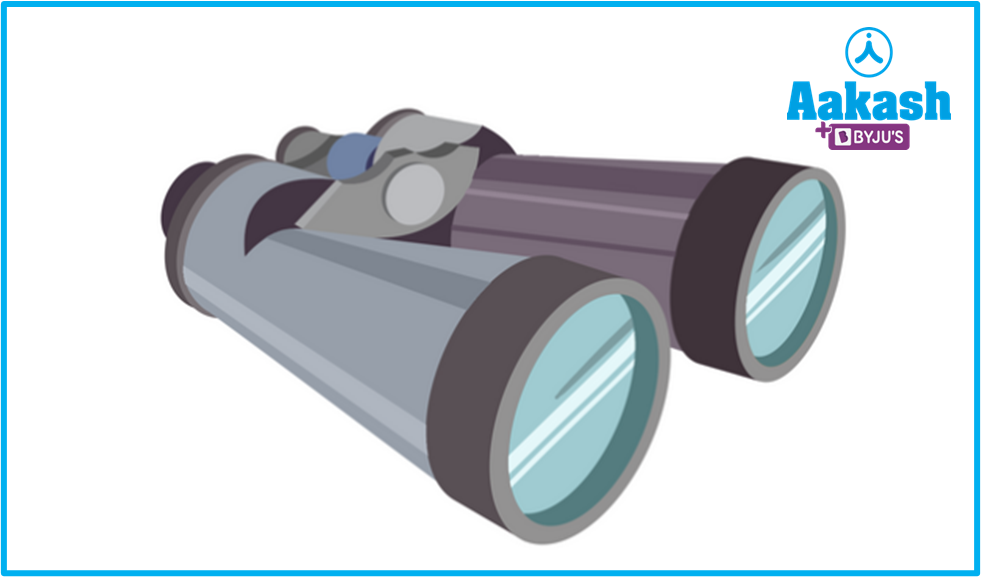## Practice problems

Q. How can a convex lens burn a piece of paper ?
A.
To burn a paper or any object, we need more heat at the point where we want a fire. The light rays coming from the sun have the energy distributed over the area. Now if we concentrate these rays at a point so that the energy associated with them is also concentrated, the paper will burn. We use the convex lens to concentrate the rays and put the paper at the focus of the lens. This results in burning of paper.

Q. How are the uses of convex lenses different from the uses of convex mirrors?
A.
The convex lens is majorly used to converge the light rays. Wherever we need converging light we can use convex lenses such as a microscope, telescope, projector etc.

Whereas the convex mirror produces virtual and small images. Since they have a wide field of view, they are generally used in side mirrors in the car or on the corner of the road to see the other road.

Q. What are some uses of concave lenses?
A.
Concave lenses have the properties to diverge the light rays. Concave lens is used in various applications where a diverging light is needed such as : concave lenses used to correct the myopia defect of the eye, it is used in the flash light so that the light coming from the torch can cover a large area.

Q. How many types of convex lenses are there? Can all of them be used as Converging lenses?
A.
There are three types of convex lens : 1. Dual convex lens 2. Plano-convex lens 3. Concavo -convex lens. They will be used as a converging lens or not will depend upon the radius of curvatures of the lens and can be found by lens maker formula.

## FAQs

Q. What is Hypermetropia?
A.
It is a defect of the human eye. In this defect a person can not see the nearby objects but can see the objects at a distance.

Q. Why is the objective convex lens used in a microscope smaller than an eyepiece ?
A.
In a microscope the magnification is inversely proportional to the focal length of the objective, so objective lenses are kept small.

Q. Why is the objective convex lens used in a telescope kept bigger than an eyepiece?
A.
Objective convex lens is kept bigger so that it can pass maximum light through objective.

Q. What is the nature of focal length of the convex lens?
A.
Focal length of the convex lens is considered positive.Talk to our expert
Resend OTP Timer =
By submitting up, I agree to receive all the Whatsapp communication on my registered number and Aakash terms and conditions and privacy policy